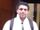## Find letter values in variables

Hi.. I am trying to come up with a SAS code that can identify all letter values among all variables. For example, I have a dataset that have 3 CHAR variables (var1 - var3):

var1    var2    var3

01       10        M9

02       3M       00

N                    09

07       18        11

How can I just extract all the letter values (values that have letter in them)?

var1    var2    var3

N         3M       M9

or, even better, how I can have output like this?

special_value

N

3M

M9

9 REPLIES 9novinosrin
Tourmaline | Level 20

## Re: Find letter values in variables

``````data have;
input (var1    var2    var3) (\$);
cards;
01       10        M9
02       3M       00
N         .           09
07       18        11
;

data want;
set have;
array t var1-var3;
do i=1 to dim(t);
if anyalpha(t(i)) then
do;
want=t(i);
output;
end;
end;
keep want;
run;
``````

## Re: Find letter values in variables

If I have 100+ variables there, can I do this?

``````%let varlist = %str(var1 ... var1000); (?)data want;
set have;
array t &varlist.;
do i=1 to dim(t);
if anyalpha(t(i)) then
do;
want=t(i);
output;
end;
end;
keep want;
run;``````novinosrin
Tourmaline | Level 20

## Re: Find letter values in variables

Sure.

I wonder why you need to quote though with %str

I believe just

%let arraylist=var1 var2 var3 varN;

should work fine

Macro quoting is not needed in this instance.

## Re: Find letter values in variables

Thank you! Let me try this! 😃

## Re: Find letter values in variables

Alternatively with regular expressions

``````data want(where=(newvars ne ''));
set have;
array vars(*) \$ var1 var2 var3;
do i = 1 to dim(vars);
newvars=ifc(prxmatch('m/[A-Z]/',strip(vars(i))),strip(vars(i)),'') ;
output;
end;
run;``````
Thanks,
Jag

Thanks! 😃

## Re: Find letter values in variables

If you truly have 100 variables wouldn't you also need to know which variable and which observation contains the character?

## Re: Find letter values in variables

Sure.. how can I do that?

## Re: Find letter values in variables

Let's build on your earlier program:

``````%let varlist = var1 ... var1000;

data want;
set have;
array t &varlist.;
do i=1 to dim(t);
if anyalpha(t{i}) then do;
want=t{i};
recno = _n_;
varname = vname(t{i}) ;
output;
end;
end;
keep want recno varname;
run;``````
Discussion stats
• 9 replies
• 580 views
• 3 likes
• 4 in conversation